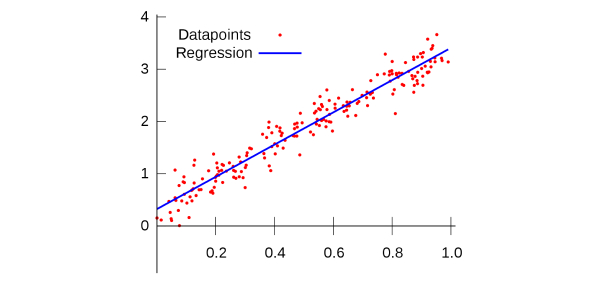Statistics Math Quiz

10 Questions | Total Attempts: 276SettingsQuiz Description

• 1.
Consider the following back-to-back stemplot: Which of the following are true statements? I.  The distributions have the same mean. II.  The distributions have the same range. III.  The distributions have the same standard deviation.
• A.

II

• B.

I and II

• C.

I and III

• D.

I, II and III

• E.

II and III

• 2.
The parallel boxplots show monthly rainfall summaries for a small village in India.   Which month has the least variation as measured by the interquartile range?
• A.

January

• B.

February

• C.

March

• D.

April

• 3.
Which of the following distributions has the smallest standard deviation?
• A.
• B.
• C.
• D.
• E.

Not possible to determine with the given information

• 4.
Following is a histogram of ages of people applying for a particular high school teaching position. Which of the following statements are true? I.  The median age is between 24 and 25. II.  The mean age is between 22 and 23. III.  The mean age is greater than the median.
• A.

I

• B.

II

• C.

III

• D.

All are true

• E.

None is true

• 5.
Following is a cumulative probability graph for the number of births per day in a city hospital. Assuming that a birthing room can be used by only one woman per day, how many rooms must the hospital have available to be able to meet the demand at least 90 percent of the days?
• A.

5

• B.

10

• C.

15

• D.

20

• E.

25

• 6.
If the test scores of a class of 30 students have a mean of 75.6 and the test scores of another class of 24 students have a mean of 68.4, then the mean of the combined group is
• A.

72

• B.

72.4

• C.

72.8

• D.

74.2

• E.

None of these

• 7.
If the largest value of a data set is doubled, which of the following is NOT necessarily true?
• A.

The mean increases.

• B.

The standard deviation increases.

• C.

The interquartile range increases.

• D.

The range increases.

• E.

The median remains unchanged.

• 8.
For the following data set, what is the upper fence for determining outliers? 1, 3, 4, 5, 5, 6, 8, 8, 10
• A.

6.75

• B.

8

• C.

10

• D.

11.75

• E.

14.75

• 9.
You want to use a five-number summary of a univariate data set, (10, 15, 25, 45, 95), to construct a (modified) boxplot. The length of the left-side whisker is 5. Using the accepted rule for determining outliers, what is the maximum length of the right-side whisker?
• A.

30

• B.

35

• C.

45

• D.

50

• E.

Cannot be determined from the information given.

• 10.
Determine the mean and standard deviation for the data set represented by the following frequency table.
• A.

32, 5.4

• B.

32, 6.3

• C.

32.7, 5.5

• D.

32.7, 5.7

• E.

33, 6

Related TopicsBack to top